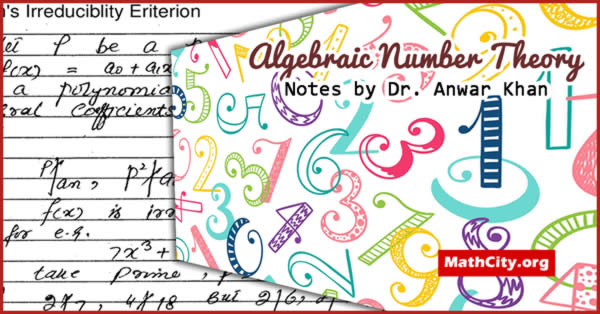# Algebraic Number Theory Notes by Anwar KhanAlgebraic number theory is a subfield of number theory that studies integers, rational numbers, and their generalisations using abstract algebra techniques. It covers Galois theory, ideals and units in rings of integers, unique factorization, and algebraic number fields and related rings of integers. It is a complex and in-depth subject with numerous linkages to other branches of mathematics.

These notes are provided by Mr. Anwar Khan. We are very thankful to him for providing these notes.

 Name Algebraic Number Theory: Notes Anwar Khan 110 pages PDF 2.8 mB
• Algebraic Number Theory
• Diophantine Equation and Fermat’s Conjecture
• Polynomial over the Rational
• Degree of Polynomial
• Monic Polynomial
• Division of Polynomial
• Irreducible Polynomial
• Division Algorithm
• Greatest Common Divisor
• Algebraic Number
• Degree of Algebraic Number
• Minimal Polynomial
• Conjugates of an Algebraic Number
• Primitive Polynomial
• Product of Polynomial
• Symmetric Polynomial
• Alternative Statement
• Primitive Element
• Eisenstein’s Irreducibility Eriterion
• Algebraic Integer
• The Determinant
• Euclidian Domain
• Square Free Rational Integer
• Prime
• Ideal
• Principal Ideal
• Congruence of an Ideal
• Norm f an Ideal
• Decrement
• Units and Primes in $\mathbb{R}$
• Unique Factorization Domain
• Arithmetic of an Ideal
• Prime Ideal
• Equivalent Ideal
• Cyclotomic Field KP
• Pure Cubic Field

• notes/algebraic-number-theory-notes-anwar-khan